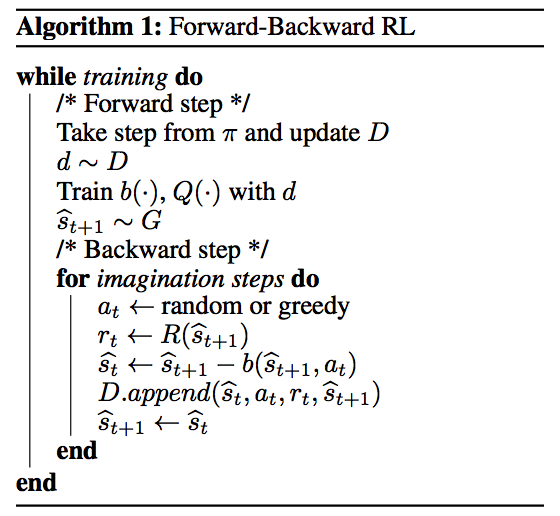# Forward-Backward Reinforcement Learning

## WHY?

Reinforcement learning with sparse reward often suffer from finding rewards.

## WHAT?

Forward-Backward Reinforcement Learning(FBRL) consists of forward and backward process. Forward process is like normal rl using memory to update Q function. In backward process, new model is introduced called backward model b. b is a neural network that predict the difference in state given action and next state.\ b(s_{t+1}, a_t)\rightarrow \hat{\Delta}$b(s_{t+1}, a_t)\rightarrow \hat{\Delta}$
Rewarded states are sampled from G which is a distribution of goal states. Using backward model, estimate the previous state and actions are sampled randomly or greedily. This imagined data is appended to the memory. Algorithm is as follows.## So?

FBRL learned much faster than DDQN in Gridworld and Towers of Hanoi environment.

## Critic

Sampling from goal states maybe difficult and backward model may be noisy in stochastic environment. Is it worth to double the parameter of model? However, the concept to take advantage of memory seems like good idea to develop.

Powered by aiden